__>>_ >> > __-- K A R S T A -- L I P P _ ________________________________________________________________________________________________________________

M A L E R E I
F O T O G R A F I E
V I T A
K O N T A K T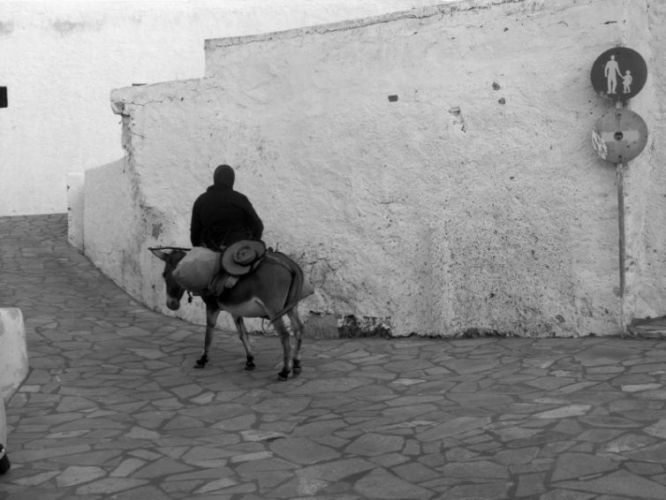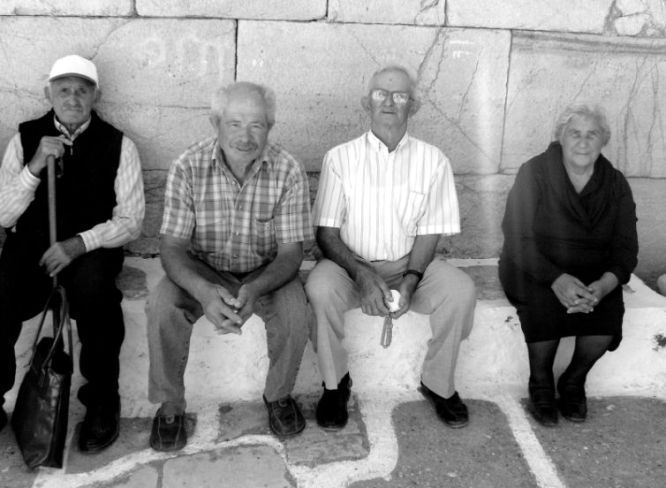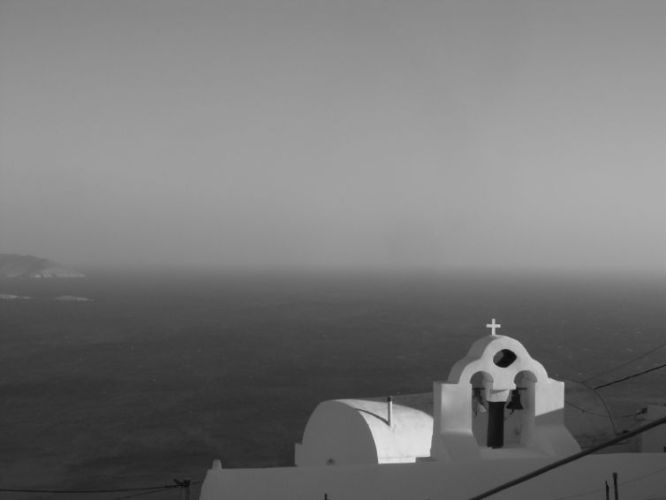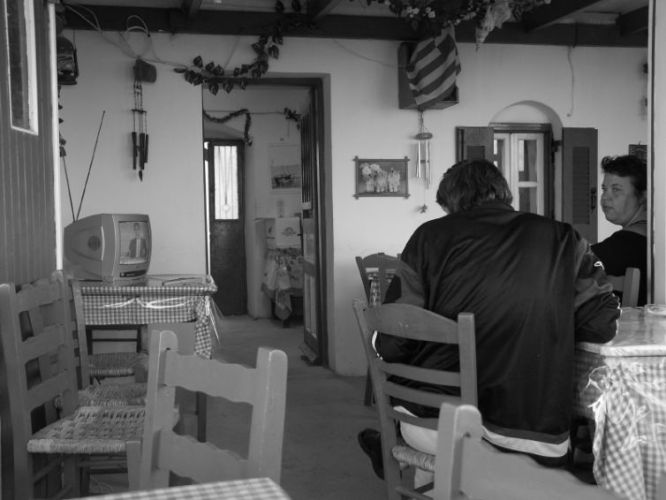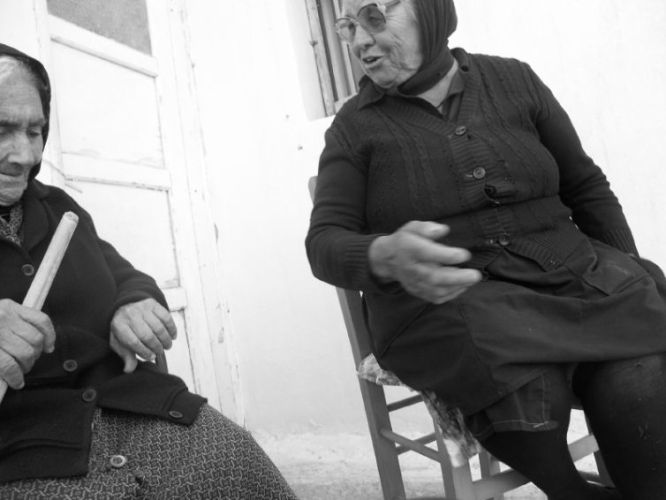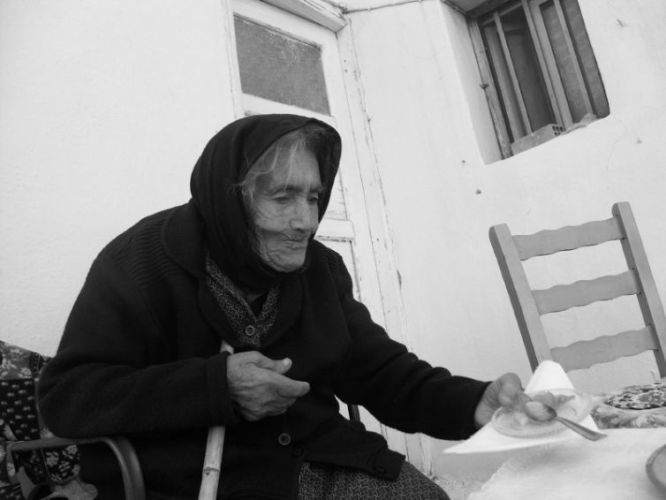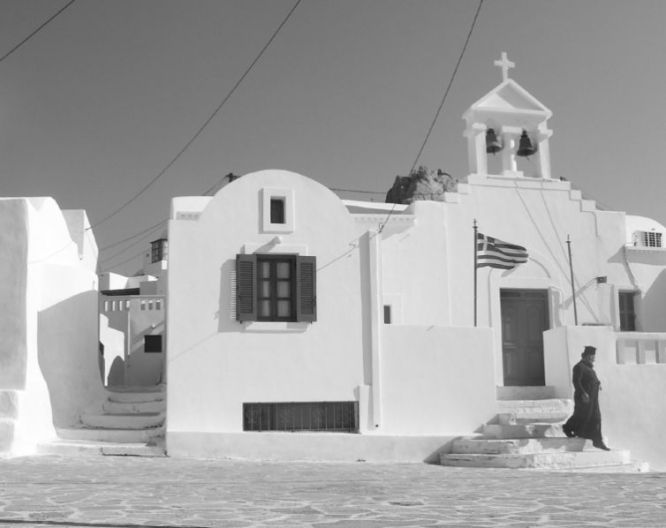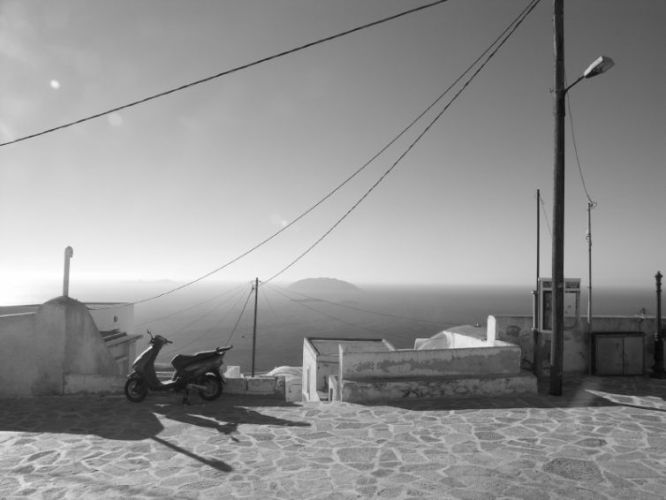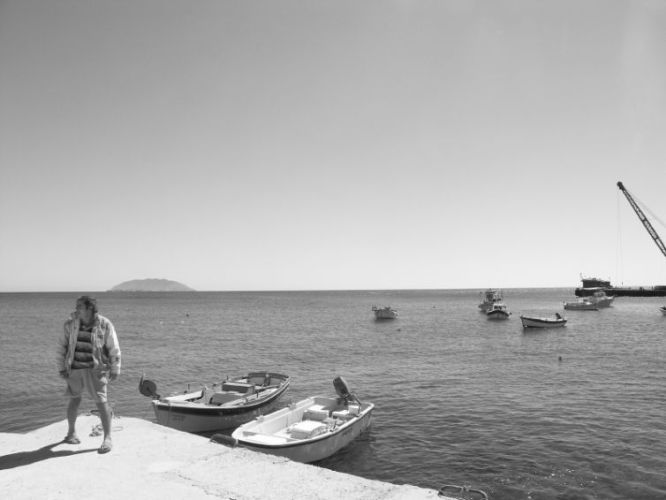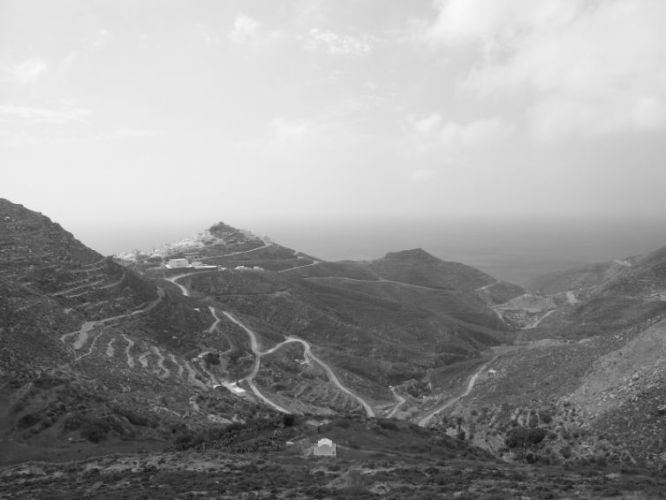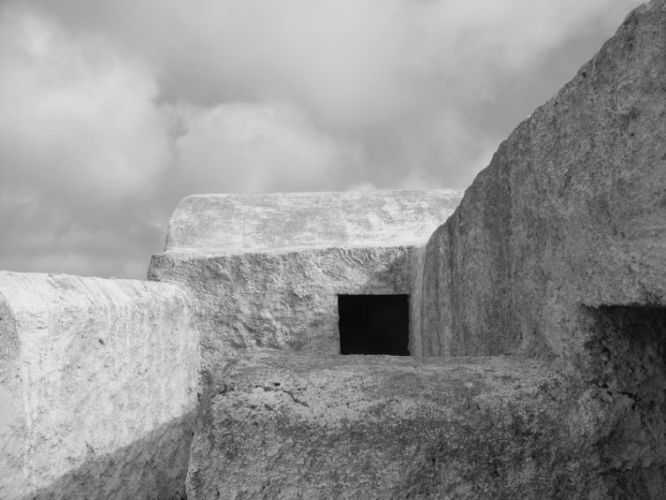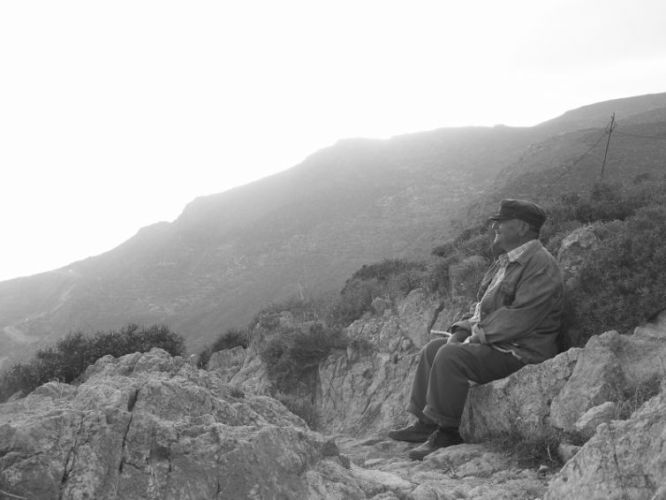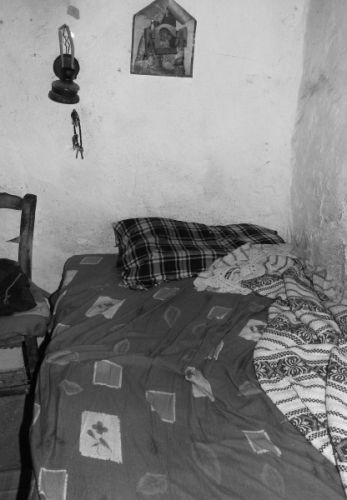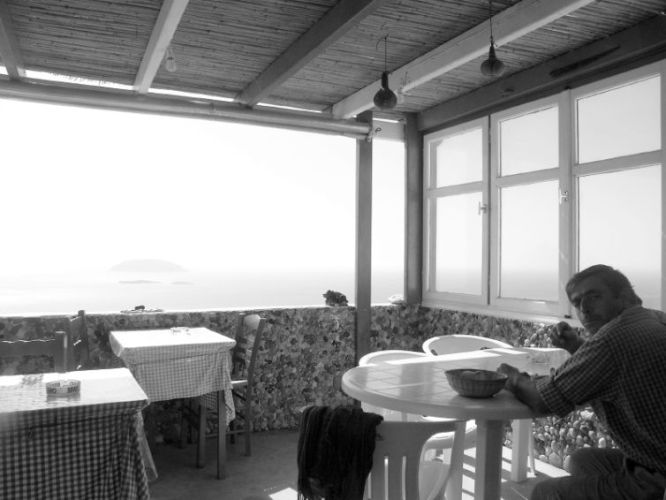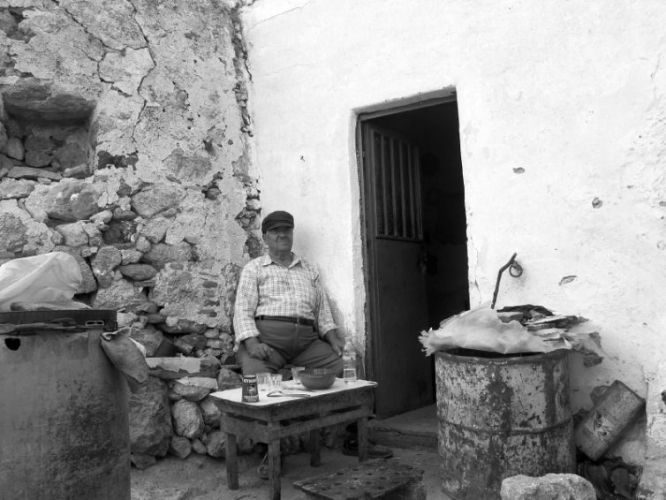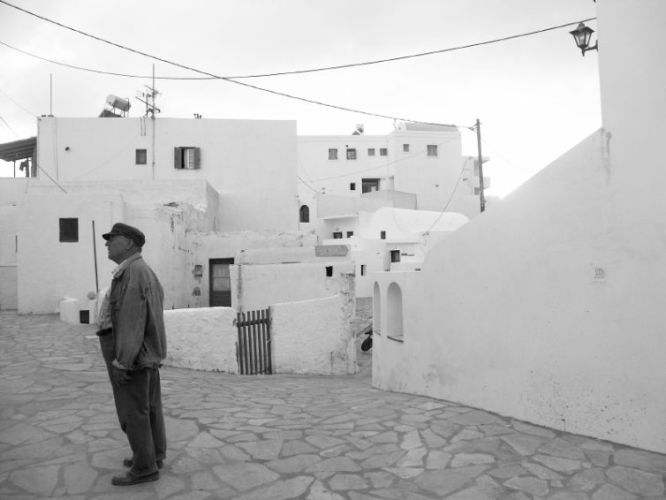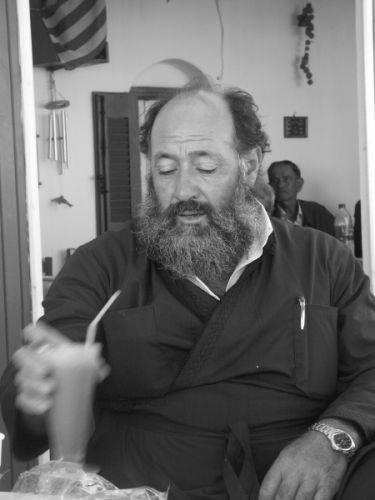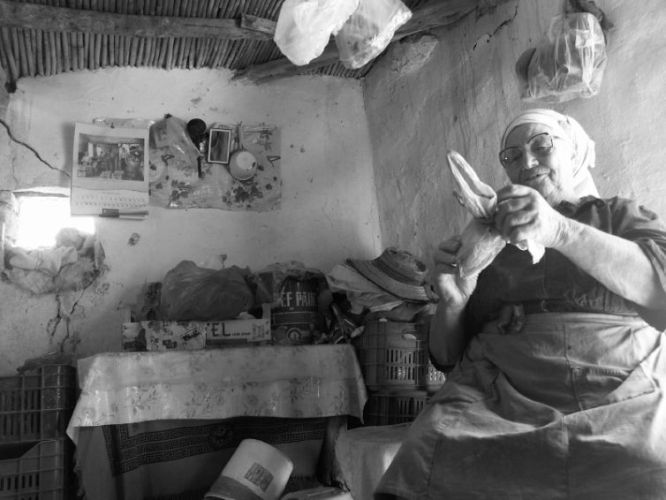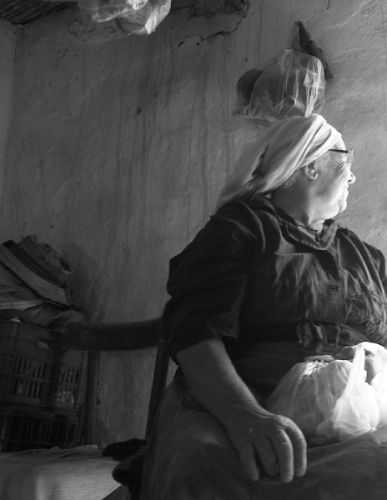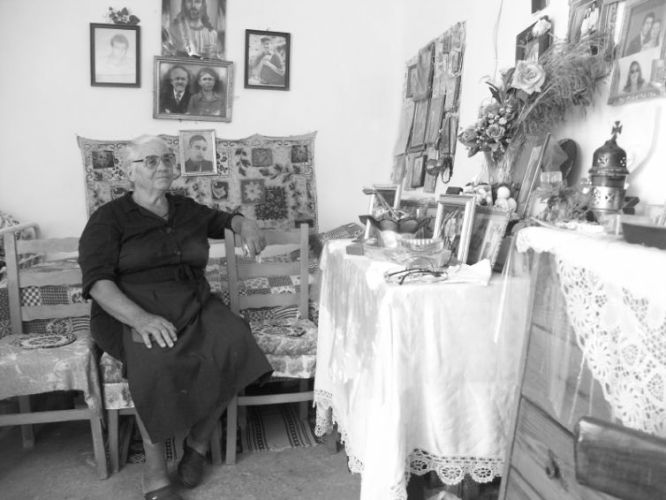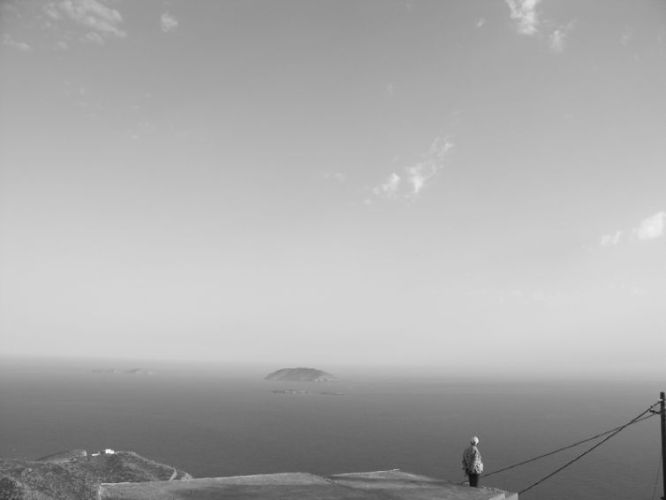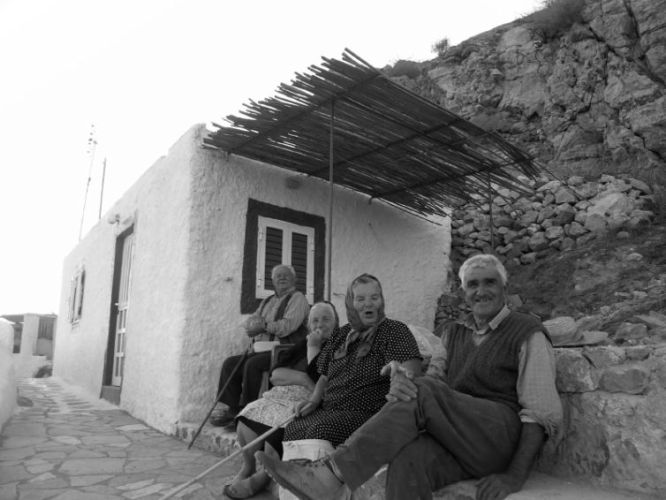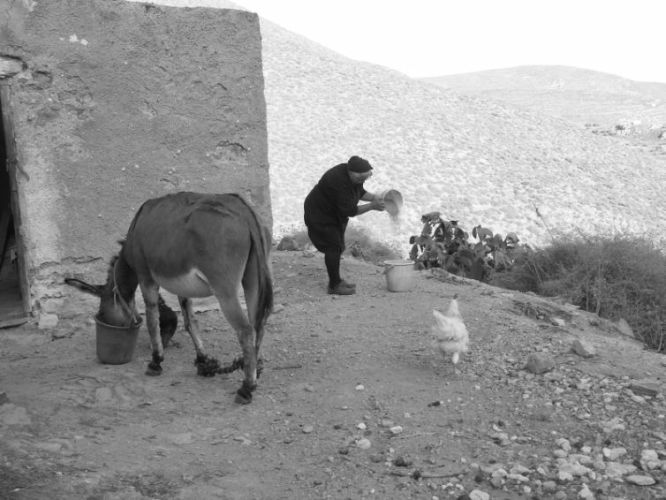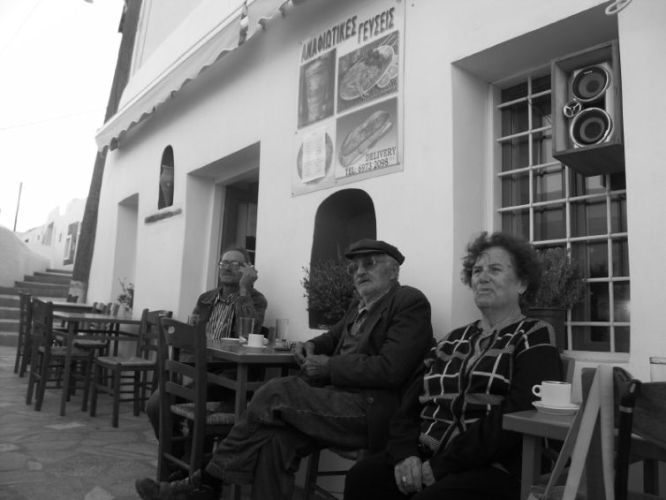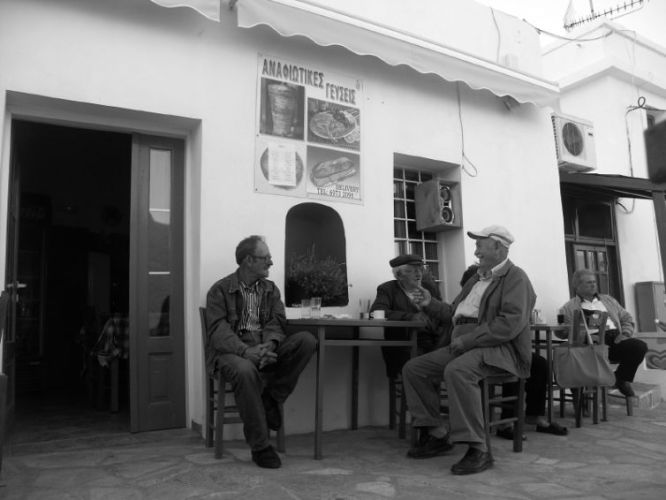<< ZURÜCK // F A R B S E R I E >>

F O T O S E R I E N

A N A F I

M E M O R I E S

M A R O K K O
G R I E C H E N L A N D

Fotoserie schwarz/weiss "Anafi"